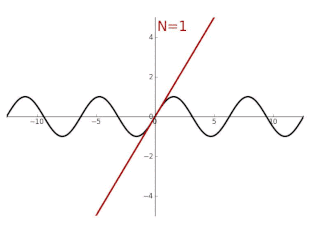# Question regarding notation when omitting terms

kent davidge
If we want to expand a function ##f(x)## up to first order around ##x = 0## say, we usually write ##f(x) = f(0) + (df/dx)|_0 x + \mathcal O(x^2)##.

But what if I want to expand ##f(x)## in the whole series, and showing only the first order term in x? What notation do you use for that? (Aside from ##f(x) = f(0) + (df/dx)|_0 x + \sum \frac{d^{k-2}f}{dx^{k-2}}x^{k-2} / (k-2)!##.)

My thought: ##f(x) = f(0) + (df/dx)|_0 x + \mathcal O(x^{\geq 2})##

Homework Helper
Hi,
if I want to expand ##f(x)## in the whole series
You don't want that, because it doesn't make sense. The only term of interest around zero is the ##x^2## term. All higher order terms in ##f(x) - f(0) - (df/dx)\Big |_0 \; x \ ## vanish for ##x\downarrow 0##.

In other words, ##\mathcal O(x^{2}) ## is the same as your ##\mathcal O(x^{\geq 2})## (or: implies it).

•kent davidge
Mentor
x3 < x2 for small x, therefore ##ax^3 \in O(x^2)##, same for all higher orders.

•kent davidge
kent davidge
x3 < x2 for small x, therefore ##ax^3 \in O(x^2)##, same for all higher orders.
I'm not considering only small ##x## though

Homework Helper
Doesn't matter. The meaning doesn't change:
$$f(x) - f(0) - (df/dx)\Big |_0 \; x \ = \mathcal O (x^2) \equiv \lim_{x \downarrow 0 }{f(x) - f(0) - (df/dx)\Big |_0 \; x \over x^2} \ \ \text {is finite}$$no more, no less. If you want to consider huge ##x## is upon you -- it probably won't be a good approximation, though.( from wikipedia sin )

•pbuk and kent davidge
Mentor
For large x you have to choose between physics and computer science convention of the notation, but in the latter case considering the constant and linear term is irrelevant and you'll likely need a completely new approach.

•kent davidge